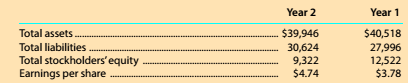Chapter 8, Problem 8.8.6MBA

Chapter
Section
Textbook Problem

Debt and price-earnings ratios The Home Depot. Inc. (HD) operates over 2.200 home improvement retail stores and is a competitor of Lowe's (LOW). The following data (in millions) were adapted from recent financial statements of The Home Depot.With a market price of \$104.43, compute the price-earnings ratio for Year 2.

To determine

Concept Introduction:

Price Earnings Ratio:

The price earnings ratio shows the relationship between price of the share and earnings per share. It is calculated with the help of following formula:

Price Earnings Ratio=Market price per shareEarnings per share

To Calculate:

The Price Earnings ratio for year 2

Explanation

The Price Earnings ratio for year 2 is calculated as follows:

Price Earnings Ratio=Market&#

Still sussing out bartleby?

Check out a sample textbook solution.

See a sample solution

The Solution to Your Study Problems

Bartleby provides explanations to thousands of textbook problems written by our experts, many with advanced degrees!

Get Started